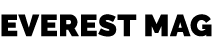Tech Matra

Authentic & Useful Insights, News, Life Style Tips

## Categories

### Everest NewsUt interdum risus felis, eget rhoncus sem aliquam nec. Sed eu congue arcu. Duis ultricies orci nec diam malesuada accumsan. Aliquam pulvinar pulvinar orci, nec ornare ex efficitur ac. Proin quis laoreet quam. Praesent sagittis mollis turpis tempus sodales. Ut efficitur tortor nec condimentum ornare.

## The Easiest way to Understand Statistics for Data Science

Today you will learn about how someone can learn statistics for data science. When we hear the word statistics, we always think it is very hard to understand. In my experience, it is not that difficult to understand. Let me tell you why.

It only requires some hard work and understanding of basic concepts. When we talk about data science, there are a lot of blogs you have already seen, but trust me, if I can do this, you’ll definitely do this. Whether you are from a technical background or a non-technical background, learning statistics for data science is for everyone.

In the initial stages of learning statistics for data science, it will be quite confusing, but after understanding the basic concepts, it will be very easy to learn.

Let’s move on to another important aspect of Data science- Python. Python is a user-friendly and easy programming language that is very easy to understand. For data science, python is a very powerful language. The main thing is that you are going to use Python for statistics implementation for data science. So, you don’t need to calculate the stats problems by yourself, you just need to understand the concept so that you can understand what you are going to do.

Let us look at some of the basic concepts that you must understand as a beginner in data science and statistics.

1. Descriptive statistics
2. Basic understanding of distribution and plots
3. Introduction to probability
4. Bayes theorem
5. Binomial and normal distribution
6. Poisson probability function

First of all, you are going to learn what descriptive statistics is. There are some techniques like, mean, median, mode and range etc.

Mean -> Average value of any sequence e.g., add all the numbers and divide by the total number. For example {4,5,6,7,8},

Mean = (4+5+6+7+8)/5

Mean = 23.6

Median -> The middle number of any sequence, first arrange the number in ascending order and mid value is your median. If the number of sequences is even then there are two middle values in this case, take the average of both middle values. For example {4,5,6,7,8}, here the median value is 6.

Mode -> The most frequent number in the sequence. For example {1,2,3,4,4,5,6,7,6,4}, here the mode is 4 which is the most occurrence value in the sequence.

Range -> This is the difference between highest value and lowest value. These are some descriptive statistics techniques.

Now, come to the probability,

Probability means possibility, it is the part of mathematics that deals with the occurrence of a random incident. The value lies between 0 to 1.

Mathematically,

Probability of event to occur P(e) = Number of favorable outcomes/Total number of outcomes

For example, a coin is tossed, there two possibilities to occur head and tail, P(head) = ½ and P(tail) = ½.

Now moves to bayes theorem,

Bayes’ Theorem is one of the strongest concepts in statistics. It is very important to know for data science professionals.

Bayes’ Theorem says the probability of occurrence of an event related to any condition. It is also applicable for the conditional probability.

Mathematically,

P(A/B) = (P(B/A) * P(A))/P(B)

Where, P(A/B) = The probability of A being true given that B is true P(B/A) = The probability of B being true given that A is true

P(A) = The probability of A being true

P(B) = The probability of B being true

Normal distribution -> The normal curve is a bell-shaped, symmetrical graph with an infinitely long base axis. The mean, median, and mode all lie at the same point (at center). A value is claimed to be normally distributed if its histogram is the shape of the traditional curve. The probability that a normally distributed value will lie between the mean and some Z-score is the area under the curve from 0 to Z (and lies between –3 to 3).

For better understanding refers to the below image.

Fig.no.1Normal distribution curve

Binomial distribution -> Binomial distributions are often used in quality control systems, where a small number of samples are tested out of a large sample, and the total sample is only accepted if the amount of defects found among the sampled units falls below a specified number. In order to analyse whether a sampling plan effectively screens out samples containing a great number of defects and accepts samples with very few defects, one must calculate the probability that a sample will be accepted given various defect levels.

These are some basic explanations about statistics for data science and don’t worry about its typical definitions once you are going to study them, they will be easy for you.